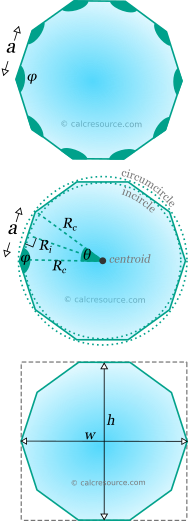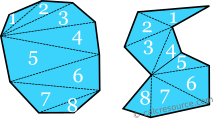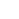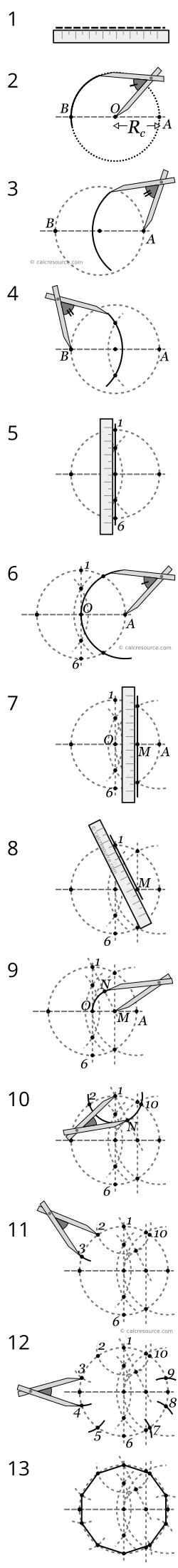## Regular decagon calculator

This tool calculates the basic geometric properties of a regular decagon. Regular polygons are equilateral (all sides equal) and also have all angles equal. The tool can calculate the properties of the decagon, given either the length of its sides, or the inradius or the circumradius or the area or the height or the width. Enter below the shape dimensions. The calculated results will have the same units as your input. Please use consistent units for any input.

 Known data: Side length Inradius Circumradius Area Height Width Geometric properties: Area = Perimeter = α = Ri = Rc = ADVERTISEMENT Bounding box: Height h = Width w = Angles : deg rad Interior φ = Central θ =## Theoretical background

### Definitions

Decagon is a polygon with ten sides and ten vertices. A decagon, like any other polygon, can be either convex or concave, as illustrated in the next figure. A convex decagon has none of its interior angles greater than 180°. To the contrary, a concave decagon (or polygon) has one or more of its interior angles greater than 180°. A decagon is called regular when its sides are equal and also its interior angles are equal. Having only the sides equal is not adequate, in that matter, since the decagon may be concave, with equal sides. As demonstrated in the figure below, many possible concave decagons can be defined, with equal sides, but unequal interior angles. These decagons are called equilateral. Any decagon that is not regular is called irregular.

The sum of the internal angles of any decagon, either convex or concave is always 1440°. This can be easily proved by counting how many triangles can be fitted inside the decagon. To find this out, we have to draw straight lines between all the vertices, avoiding any intersections. The result is exactly eight triangles. Even through, the vertices can be connected in a different manner (resulting in different triangles), the number of triangles remains constantly eight. The sum of internal angles in one triangle is 180°, therefore for 8 triangles, side by side, the total angles should measure up to 8x180=1440°.The diagonals of an decagon separate its interior into 8 triangles

### Properties of regular decagons

#### Symmetry

There are ten axes of symmetry in a regular decagon. Five of them pass through diagonally opposite vertices and the remaining five, pass through the midpoints of opposite edges. All the axes meet at a common point, the center of the decagon.

#### Interior angle and central angle

Regular decagons have equal interior angles, by definition. Since there are ten angles, in total, each one of them should be equal to:

The central angle , is the angle between two successive diagonals of the regular decagon (lines that connect opposite vertices). There are ten central angles, totally, around the common center of the decagon (where all the diagonals intersect), therefore each central angle, , should be:

In the internal triangle, that is formed by two successive diagonals and the edge of decagon, the two angles adjacent to the edge, are equal to 72°. These are indeed , because the diagonals of the decagon are also axes of symmetry, thus bisecting the interior angles (which are 144°).

The interior and central angles are supplementary, since their sum is 180°:

This property is common for all regular polygons.The regular decagon is composed by ten identical isosceles triangles having a common vertex, the polygon center.

#### Circumcircle and incircle

A circle can be drawn that passes through all ten vertices of the regular decagon. This is the cirmuscribed circle or circumcircle. The center of this circle is also the center of the decagon. The diagonals of the regular decagon that connect opposite vertices are diameters of the circumcircle. The radius of circumcircle is usually called circumradius

Another circle can also be drawn, that passes through the midpoints of all ten decagon edges. This circle is called inscribed circle or incircle. The radius of incircle is usually called inradius. The incircle is tangent to all ten edges and share the same center with the circumcircle.

All regular polygons feature a circumcircle and an incircle.

The circumcircle and incircle radii, and , respectively, are related to the edge length, . The relevant relationships can be found, using the right triangle, highlighted in the next figure, with these sides: the circumradius, the inradius and half the decagon edge. Using basic trigonometry we may find:

where the central angle and the edge length. These expressions, are valid for any regular polygon, not just the decagon. Specific expressions for the regular decagon can be derived by setting θ = 36°, the value of the central angle. The resulting expressions are:

.

Also, approximations for the circumradius and inradius of the decagon, are given by the following formulas:

#### Area and perimeter

The total area of a regular decagon can be separated into 10 identical isosceles triangles, as indicated in the figure below. The height of any of these triangles, perpendicular to the decagon edge , is indeed a radius of the incircle, therefore its length is equal to . Therefore, the area of each triangle should be , and as a result, the total area of the eight triangles becomes:

An approximate expression for regular decagon area is:

The perimeter of any N-sided regular polygon is simply the sum of the lengths of all edges: . Therefore, for the regular decagon, with 10 edges:

### Bounding box

The bounding box of a planar shape is the smallest rectangle that encloses the shape completely. The dimensions of this rectangle are defined by the height and the width . Intuitively, the bounding rectangle of the regular can be identified, as shown in the next figure:

##### Height

The height of the regular decagon is the distance between two opposite edges. This is equal to twice the inradius :

In order to relate the height with the circumradius , or the side length , the respective analytical expressions for these quantities should be employed. The following formulas are then derived:

which are approximated to:

##### Width

The width is the distance between two opposite vertices of the regular decagon (the length of its diagonal). This is equal to twice the circumradius :

Expressed in terms of edge length the width becomes:

which is approximated by:

### How to draw a regular decagon

A regular decagon,can be constructed, using only a ruler and a compass, given its circumradius . The procedure is described by the following steps and illustrated in the figure below:

1. First just draw a line (a bit larger than the wanted diameter of the circumcircle).
2. Mark two points on the line: one is the center of the polygon (point O), and the other is a point of the circumcircle (point A). The distance OA is the circumradius . Draw a circle around the center point, that passes through A. This is the circumcircle. Mark another point where the circumcircle intersects with the line (point B).
3. Then construct a circular arc, having its center at point A, and radius a bit larger than .
4. Also a circular arc, having its center at point B, and same radius with step 3. Mark the two points, where the two arcs intersect.
5. Draw a line, passing through the intersections of the two arcs from the last step (also passing through the center point O) and mark two more points, 1 and 6, where this line intersects with the circumcircle.
6. Place the tip of the compass on point A and construct a new circular arc, passing through center point O. Mark the two points the arc intersects with the circumcircle.
7. Draw a line, passing through the two intersection points from the previous step. Mark point M, where this line crosses with linear segment OA.
8. Draw a line, from point 1 to point M.
9. Place the tip of the compass on point M and draw a circular arc, passing through center point O. Mark point N, where this arc intersects with the line drawn in the previous step.
10. Placing the tip of the compass on point 1, draw a circular arc, passing through point N. Mark the two points, 2 and 10, where the arc intersects with the circumcircle.
11. Using the same radius, draw a circular arc, centered around point 2, and mark point 3, where the arc intersects with the circumcircle.
12. Repeat the last step, this time, using point 3 as center of the arc. As a result, point 4 is defined at the intersection with the circumcircle. Continue this way, drawing arcs and marking points around the circumcircle.
13. Ten points should have been marked so far around the circumcircle, 1 to 10. These are the vertices of the decagon. Draw linear segments between them and the regular decagon is now complete.

The following figure illustrates the drawing procedure step by step.The figure illustrates how to draw a regular decagon using a ruler and a compass.

### Examples

##### Example 1

Determine the circumradius, the inradius and the area of a regular decagon with edge length .

The circumradius and the inradius, in terms of the edge length , for the regular decagon, have been derived in the previous sections. These are:

Therefore, we have to simply substitute . Doing so we find the circumradius:

The area of a regular decagon is given by the formula:

Substituting we find:

##### Example 2

Calculate the edge length for the following regular decagons:

1. having area
2. having height
##### 1. Regular decagon with given area

The area of a regular decagon , in terms of the side length , is given by the equation:

Since we are looking for , we have to rearrange the formula:

From the last equation we can calculate the required edge length , if we substitute :

.

##### 2. Regular decagon with given height

The height of the regular decagon is related to the side length with the equation:

Rearranging:

Then we can calculate the required edge length , if we substitute :

.

##### 3. Regular decagon with given circumradius

The circumradius of the regular decagon is related to the side length with the formula:

Therefore:

From the last equation we can calculate the required edge length , if we substitute :

.

### Regular decagon cheat-sheet

In the following table a concise list of the main formulas, related to the regular decagon is included. Also some approximations that may prove handy for practical problems are listed too.